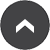# Year 3 - Miss Bossman

## Maths - Reasoned Rounding

23 Oct 2015

This week we played a Reasoned Rounding Game to practice rounding. (Nrich)

The first player rolls a 0-9 die twice and chooses which two-digit number they would like to make from the numbers rolled.

They then have to round this to the nearest multiple of 10, find the matching circle on the recording sheet and write their two digit number in one of the spaces in that circle.

Player two rolls the die twice to take their turn in a similar way.

A circle is complete when it has two numbers in it and this scores a point for the person who wrote the second number in. (Even if the first number was written in by the other player.)

The game is over when all the circles are full and then the points are counted up to find the winner.Mmmmm... does this number round to the next or previous multiple of 10?43 is a 2 digit number.
There is a 3 in the Units Column.
This means that 43 will round to the previous multiple of 10, which is 40.I rolled the dice twice.
The 2 digits are 4 and 5.
I am going to make the number 45.
Because there is a 5 in the Units Column,  45 is rounding to the next multiple of 10.Yes, I agree with you.It is your turn to roll the dice.I am going to use the 6 in the Tens Column.Yes, 26 rounded to 30.Go to the page-top